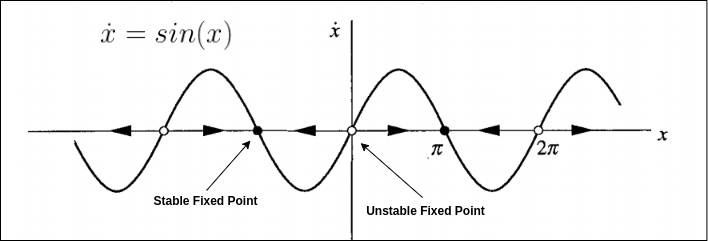# Stablility of Fixed Point of a Dynamical System

Published:

Stability theory is used to address the stability of solutions of differential equations. A dynamical system can be represented by a differential equation. The stability of the trajectories of this system under perturbations of its initial conditions can also be addressed using the stability theory.

## Fixed Point

Consider a dynamical system given by the following ordinary differential equation (ODE):

$\dot x = f(x)$

A fixed point of this system is given by:

$\dot x = 0$

Therefore, $f(x) = 0$ or roots of the function $f(x)$ form the fixed points of the dynamical system.

## Stable and Unstable Fixed Points

In layman’s terms, you can say the following about stable and unstable fixed points.

Stable Fixed Point: Put a system to an initial value that is “close” to its fixed point. The trajectory of the solution of the differential equation $\dot x = f(x)$ will stay close to this fixed point.

Unstable Fixed Point: Again, start the system with initial value “close” to its fixed point. If the fixed point is unstable, there exists a solution that starts at this initial value but the trajectory of the solution will move away from this fixed point.

In other words, one can also think of a stable fixed point as the attractor and unstable fixed point as the repeller. A particle governed by $\dot x = f(x)$, a stable fixed point will force this particle to move towards itself. On the other hand, an unstable fixed point will force a particle away from itself.

### Mathematical Intuition:

For a dynamical system, $\dot x = f(x)$, a fixed point is $f(x) = 0$.

If $f^{\prime}(x) \gt 0$, we have magnitude of $f(x)$ increasing at x. This can be represented as $f(x - \delta) \lt 0 \lt f(x + \delta)$ for a sufficiently small value of $\delta \gt 0$.

Thus, if we start from $x+\delta$ which is “close” $x$, the ODE from equation (1) will keep on increasing the value of $x$. And it we start from $x-\delta$ which is “close” $x$, equation (1) will move the particle way from $x$ by decreasing the value of $x$.

Therefore, if $f^{\prime} (x) \gt 0$, we have an unstable fixed point and vice versa.

Note: The condition of $f^{\prime} (x) \lt 0$ or $f^{\prime} (x) \gt 0$ are sufficient conditions to guarantee fixed point stability or unstability respectively. These are not the necessary conditions, i.e., it is possible to have stable and unstable fixed points where $f^{\prime} (x) = 0$.

## Intuitive Example:

For the differential equation $x^{\prime} = sin(x)$:Using linear stability analysis, fixed points occurs when $f(x)=sin(x)=0$ or $x=kπ$ where $k$ is integer.

$f^{\prime}(x)=cos(kπ)=1$ if $k$ is even. and $f^{\prime}(x)=cos(kπ)=−1$ if $k$ is odd.

Therefore, $x$ is unstable when $k$ is even, and stable if $k$ is odd.

### High Dimensional Dynamical Systems

This post discussed the definition of a fixed point of a dynamical system. A simple one-dimensional dynamical system is used as an illustration to explain the concept. A more detailed discussion on general nonlinear, continuous-time, multi-dimensional dynamical systems and their fixed points is provided in my next post.